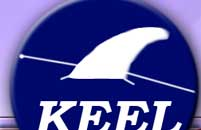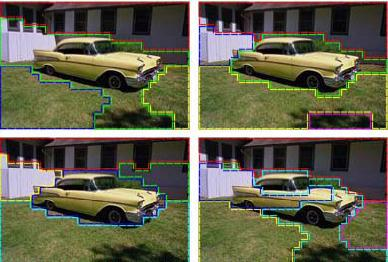KEEL-dataset - data set descriptionThis section describes main characteristics of the segment data set and its attributes:

### General information

Image Segmentation data set
TypeClassificationOriginReal world
Features 19(Real / Integer / Nominal)(19 / 0 / 0)
Instances2310 Classes7
Missing values?No

### Attribute description

AttributeDomainAttributeDomain
Region-centroid-col[1.0, 254.0]Rawred-mean[0.0, 137.11111]
Region-centroid-row[11.0, 251.0]Rawblue-mean[0.0, 150.88889]
Region-pixel-count[9.0, 10.0]Rawgreen-mean[0.0, 142.55556]
Short-line-density-5[0.0, 0.33333334]Exred-mean[-49.666668, 9.888889]
Short-line-density-2[0.0, 0.22222222]Exblue-mean[-12.444445, 82.0]
Vedge-mean[0.0, 29.222221]Exgreen-mean[-33.88889, 24.666666]
Vedge-sd[0.0, 991.7184]Value-mean[0.0, 150.88889]
Hedge-mean[0.0, 44.722225]Saturatoin-mean[0.0, 1.0]
Hedge-sd[-1.5894573E-8, 1386.3292]Hue-mean[-3.0441751, 2.9124804]
Intensity-mean[0.0, 143.44444]Output{1, 2, 3, 4, 5, 6, 7}

This database contains instances drawn randomly from a database of 7 outdoor images (classes). The images were handsegmented to create a classification for every pixel. Each instance encodes a 3x3 region.

The task is to determine the type of surface of each region.

Attributes description:

1. Region-centroid-col: the column of the center pixel of the region.
2. Region-centroid-row: the row of the center pixel of the region.
3. Region-pixel-count: the number of pixels in a region = 9.
4. Short-line-density-5: the results of a line extractoin algorithm that counts how many lines of length 5 (any orientation) with low contrast, less than or equal to 5, go through the region.
5. Short-line-density-2: same as short-line-density-5 but counts lines of high contrast, greater than 5.
6. Vedge-mean: measure the contrast of horizontally adjacent pixels in the region. There are 6, and this is the mean value. This attribute is used as a vertical edge detector.
7. Vegde-sd: the contrast of horizontally adjacent pixels in the region. There are 6, and this is the standard deviation. This attribute is used as a vertical edge detector.
8. Hedge-mean: measures the contrast of vertically adjacent pixels. Used for horizontal line detection (mean)
9. Hedge-sd: measures the contrast of vertically adjacent pixels. Used for horizontal line detection (standard deviation)
10. Intensity-mean: the average over the region of (R + G + B)/3
11. Rawred-mean: the average over the region of the R value.
12. Rawblue-mean: the average over the region of the B value.
13. Rawgreen-mean: the average over the region of the G value.
14. Exred-mean: measure the excess red: (2R - (G + B))
15. Exblue-mean: measure the excess blue: (2B - (G + R))
16. Exgreen-mean: measure the excess green: (2G - (R + B))
17. Value-mean: 3-d nonlinear transformation of RGB (see James D. Foley and Andries Van Dam. 1982. Fundamentals of Interactive Computer Graphics. Addison-Wesley Longman Publishing Co., Inc., Boston, MA, USA)
18. Saturatoin-mean: (see James D. Foley and Andries Van Dam. 1982. Fundamentals of Interactive Computer Graphics. Addison-Wesley Longman Publishing Co., Inc., Boston, MA, USA.)
19. Hue-mean: (see James D. Foley and Andries Van Dam. 1982. Fundamentals of Interactive Computer Graphics. Addison-Wesley Longman Publishing Co., Inc., Boston, MA, USA.)

In this section you can download some files related to the segment data set:

 Copyright 2004-2018, KEEL (Knowledge Extraction based on Evolutionary Learning)